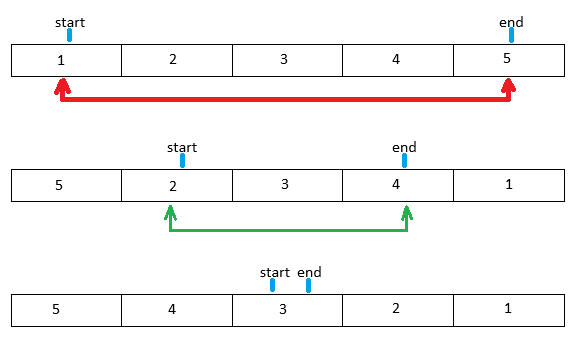# Reverse an ArrayAn array is the sequential collection of similar elements. The array is a fixed size data structure. Arrays can be accessed by an index that starts from zero. Reversing the array helps in arranging all the elements of an array-like we are reading elements from the end. We have given an array of integers and we have to reverse it or simply say reverse an array.

There are various methods to reverse an array. Below is an array as input and we have to write a program to Reverse an Array.

## Input FormatFirst-line containing an integer N which denotes the size of the array.

Second-line containing an array or simply say n integers.

## Output FormatPrint the reverse of the input array.

## Constraints• 1<=N<=1000000
• -1e9<=a[i]<=1e9

Input

1 2 3 4 5

Output

5 4 3 2 1

## Approach 1 using Two Pointer Method for Reverse an ArrayWe take two variables start (the point at the first element of an array) and end (Point at last element of an array). Reverse the element of a[start] and a[end] and then increment start by 1 and decrement end by 1. We keep moving till we hit the condition start>end. For a strong grasp on the implementation see the below algorithm:

Degree of an array

### Algorithm

1. Loop till start is less than the end.

2. Keep on swapping the elements pointed by start and end.

3. Increment start and Decrement end variable.

4. As start equals end or Greater than end then we will stop the loop.### C++ Program

```#include <bits/stdc++.h>
using namespace std;
int main()
{
int n;
cin>>n;
int a[n];
for(int i=0;i<n;i++)
{
cin>>a[i];
}
int start=0,end=n-1;
while(start<end)
{
swap(a[start],a[end]);
start++;
end--;
}
for(int i=0;i<n;i++)
{
cout<<a[i]<<" ";
}
cout<<endl;
}```
```6
1 2 3 4 5 6```
`6 5 4 3 2 1`

### Complexity Analysis for Reverse an Array

#### Time Complexity

O(N) where N is the number of elements present in the array. Here we run one loop for N/2 times.

#### Space Complexity

O(1) because we don’t use any auxiliary space we just use start and end variables to swap the array.

## Approach 2 for Reverse an Array using Recursion### Algorithm

1. Set start = 0 and end = N-1.

2. Call the reverse function which has parameters array, size of the array, start, end.

3. In each call, we check if the start is greater than the end or not.

4. If start is less than then we swap the values of a[start] and a[end] and call the reverse function by increment the value of start and decrement the value of end.

5. If the start is greater than the end then stop the process and print the final array.

Like Approach 1, we will do traversing forward and backward by swapping values. But in this case, we will use recursion. It uses extra space because of the usage of Stack.

### C++ Program

```#include <bits/stdc++.h>
using namespace std;
void reverse(int a[],int n,int start,int end)
{
if(start>end)
{
return;
}
else
{
swap(a[start],a[end]);
reverse(a,n,start+1,end-1);
}
}
int main()
{
int n;
cin>>n;
int a[n];
for(int i=0;i<n;i++)
{
cin>>a[i];
}
reverse(a,n,0,n-1);
for(int i=0;i<n;i++)
{
cout<<a[i]<<" ";
}
cout<<endl;
}```
```7
-1 5 3 -234 45622 1000000000 -32213```
`-32213 1000000000 45622 -234 3 5 -1`

### Complexity Analysis for Reverse an Array

#### Time Complexity

O(N) where N is the number of elements present in the array. Here we call reverse function N/2 times and each call we swap the values which take O(1) time.

All Unique Triplets that Sum up to a Given Value

#### Space Complexity

O(N) because we recursively call to reverse function N/2 times which means our stack size is N/2.

## Approach 3 for Reverse an Array using STL Function### Algorithm

1. Take the input in the vector variable.

2. Use STL function reverse(variable.begin(),variable.end())

3. Print the vector.

### C++ Program

```#include <bits/stdc++.h>
using namespace std;
int main()
{
int n;
cin>>n;
vector<int> V;
for(int i=0;i<n;i++)
{
int x;
cin>>x;
V.push_back(x);
}
reverse(V.begin(),V.end());
for(int i=0;i<n;i++)
{
cout<<V[i]<<" ";
}
cout<<endl;
}```
```4
-100 0 2 100```
`100 2 0 -100`

### Complexity Analysis for Reverse an Array

#### Time Complexity

O(N) where N is the number of elements present in the array. Here we use inbuild reverse() function which has O(N) time complexity.

#### Space Complexity

O(1) because we use a reverse() function which has O(1) space complexity.

This is a commonly asked question in interviews where they want to check your programming and logical skills to reverse an array. The array reverse algorithm should be efficient. Array reverse program should be written in O(n) time complexity then only your solution will be considered.